Courses

# How to prepare for Class 8 Maths: Tips & Tricks for Maths Class 8 Notes | EduRev

## Class 8: How to prepare for Class 8 Maths: Tips & Tricks for Maths Class 8 Notes | EduRev

The document How to prepare for Class 8 Maths: Tips & Tricks for Maths Class 8 Notes | EduRev is a part of Class 8 category.
All you need of Class 8 at this link: Class 8

One thing to be kept in mind all along the way for Maths preparation is that practice is the key here. The more you practice for every topic, the better understanding is achieved.

The main topics in Class 8 Maths are basic arithmetic, algebra, geometry and mensuration, probability, etc. These topics should be practiced as much as possible. The key to solve problems is to by-heart the formulas, understand the theorems, and practice as many times as possible. Maths can become your favorite subject with practice and dedication.Mathematics Class 8 (VIII) - CBSE & NCERT Curriculum

Includes 87 docs, 221 videos & 39 tests

Tips & Tricks for Mathematics :

1. Study from the Mathematics NCERT Textbook and practice all the solved examples and exercise questions thoroughly before moving forward to extra practice from additional books. Refer to NCERT Solutions in case of any doubt.

## NCERT Textbooks and solutions

Chapter 1 - Rational Numbers

Chapter 2 - Linear Equations in One Variable

Chapter 4 - Practical Geometry

Chapter 5 - Data Handling

Chapter 6 - Squares &amp; Square Roots

Chapter 7 - Cubes &amp; Cubes Roots

Chapter 8 - Comparing Quantities

Chapter 9 - Algebraic Expressions &amp; Identities

Chapter 10 - Visualising Solid Shapes

Chapter 11 - Mensuration

Chapter 12 - Exponents &amp; Powers

Chapter 13 - Direct and Inverse Proportions

Chapter 14 - Factorisation

Chapter 15 - Introduction to Graphs

Chapter 16 - Playing with Numbers

2. Practice, above all. The range of questions in class 8 is limited. With substantial practice, most question types will be internalized, and you won’t have to think for most questions.

3. You need to develop a really good understanding of all the topics included in the syllabus. It will help you with those challenging or difficult questions or derive formulae in case you forget. This can be done by watching videos for various topics that will help you develop an interest in the topic.

4. Understand all the concepts in as much depth as you can. And then solve different varieties of questions and problems. Attempt various topic-wise and chapter-wise tests. Also, practice more with sample papers and previous year papers. Use your knowledge to solve some real life questions as well.

## Practice tests

5. Give more importance to the topics which have high weightage. Solve the previous year exam papers which will increase your confidence and give you a pre-exam feel. It also helps to brush up all your concepts before the exam.

6. Don’t just read or mug up the topics but practice them. This is the most important thing which you need to keep in mind as reading maths does not help you. Instead, make a routine and give some time for practice.

Important Topics and Preparation tips for Each Chapter

Properties of Real numbers, integers, whole numbers, rational numbers and natural numbers – Commutative, associative and closure.  Roll of 1 and additive inverse of multiplication and addition. Representation of a rational number on number line and to find rational numbers between any two rational numbers. Additive identity (0) and the multiplicative identity (1).

Chapter 1: Rational Numbers

Properties of Real numbers, integers, whole numbers, rational numbers and natural numbers – Commutative, associative and closure.  Roll of 1 and additive inverse of multiplication and addition. Representation of a rational number on number line and to find rational numbers between any two rational numbers. Additive identity (0) and the multiplicative identity (1).

Chapter 2: Linear Equations in One Variable

The chapter linear equations in one variable deals with linear expression in one variable only. We know that an algebraic equations are equality involving variables. In a linear equations the values of expression on LHS are equal to values on RHS. To solve these equations, we perform the same mathematical operations on both sides of the equation, so that the balance is not disturbed. There are equations in which variable is only one side, variable is on both the side, reducing into simple equation and then to solve and applications based on linear equations.

Understanding quadrilaterals – A simple closed curve made up of only line segments is called polygon and the curves that are not closed are not polygon. Types of polygon – Triangle, quadrilateral, pentagon, hexagon. A diagonal is a line segment connecting two non-consecutive vertices of a polygon. If all sides of a polygon is equal it is a regular polygon. The sum of external angles of any polygon is 360. A parallelogram is a quadrilateral with each pair of opposite sides parallel.

Chapter 4: Practical Geometry

Practical geometry includes the constructing a quadrilateral four sides and one diagonal are given or when two adjacent sides and three angles are given or when three sides and two included angles are given, etc. Using the given specification, the quadrilateral can be drawn uniquely in this chapter.

Chapter 5: Data Handling

The collection of information to study and analyze it further about any fact/case study is called data. Organize the data in systematic way or in a required manner and then interpret it is called data handling. To get clear idea about any data it is represented graphically as a pictograph, a bar graph, double bar graph, histogram and pie chart. In this chapter, a brief discussion about probability is also given.

Chapter 6: Square and Square Roots

The square and square roots chapter gives an idea about how to find the roots of  a number. If a number, say x can be expressed as the square of any other number, say y, then y is the square root of x. Square root is the inverse operation of square. A natural number is called perfect square if its square root is a natural number. The method of finding square roots of a decimal number is frequently used even in higher classes.

Chapter 7: Cube and Cube Roots

In cube and cube roots, there are various methods to find cubes/cube roots of any number. We can get cubes of numbers by adding consecutive odd numbers pattern. Prime factorization method is also used to find cubes or cube roots. In the prime factorisation method, if every factor appears thrice, then the number is perfect cube.

Chapter 8: Comparing Quantities

Comparing quantities compares the increase or decrease percentages, marked price, sales price, discount, discounted values of the article checking whether profit or loss %. Treatment of additional expense during the purchase of an article. Sales tax and cost including or excluding the tax. Simple interest and compound interest when interest is compounded half yearly, quarterly, monthly or yearly. Comparing simple interest and compound interest on the same amount.

Chapter 9: Algebraic Expressions and Identities

This chapter gives a basic idea about algebraic expressions and identities and their implementation. Terms are added to form algebraic expression and these terms themselves can be formed as the product of factors. The numeral factor of a term is called its coefficient.  Monomials, binomials, trinomials and so on, depending the number of terms. Addition of algebraic expression taking like terms together and multiplication of polynomials by polynomials. Standard identities and their uses in solving algebraic expressions.

Chapter 10: Visualizing Solid Shapes

In visualizing solid shapes, we will study about the solid objects having length, breadth and height that is why it is called 3-D figures. For example cubes, cuboids, cones, cylinders, spheres, hemispheres, etc. are the 3-D figures. In this chapter we will try to find faces, edges and vertices of some solid figures like cuboids, triangular pyramids, triangular and square base prisms. Application of Euler’s formula F + V – E = 2, where F – Faces, V – Vertices and E – Edges.

Chapter 11: Mensuration

In mensuration chapter, we will workout the problems based on perimeter & areas of plane closed figure and surface area (sum of area of its all faces) & volume (amount of region occupied by a solid) of some solid figure like cube, cuboid, cylinder, etc. Conversion related to volume as 1 cubic cm = 1 mL, 1 L = 1000 cubic cm and 1 cubic m = 1000000 cubic cm = 1000 L

Chapter 12: Exponents and Powers

Main points – Exponents and Powers: Powers with negative exponents, scientific notation of decimal numbers, questions based on laws of exponents, use of exponents to express the numbers in standard form and comparison of very large numbers to small numbers.

Chapter 13: Direct and Inverse Proportions

Questions based on direct proportion ( when the quantity of one value increases the other also increases), inverse proportion ( if one quantity increases, the other quantity decreases and vice versa). Direct and inverse proportion can be identified on the basis of relative increase of decrease of other quantity with respect to first one. Most of the questions on this topic are interesting  and based on daily life.

Chapter 14: Factorization

Factorization of natural numbers and algebraic expression. These factors may be numbers, algebraic variables or algebraic variables or expressions. Factorization method of common factors, by regrouping terms, using identities and using factors of the form (x + a)(x + b). Division of algebraic expression – monomial by another monomial, polynomial by monomial and polynomial by polynomial. Error finding in algebraic equations.

Chapter 15: Introduction to Graphs

Introduction to graphs – The purpose of the graph (visual representations of data collected) is to show numerical facts in visual form so that every one can understand it easily, more clearly and quickly. Types of graphs – Bar graph, histogram, pie chart, line graph and a linear graph (on xy – plane). Application based on graph – Quantity & cost, principal & simple interest and time & distance using dependent variable & independent variable.

Chapter 16: Playing with Numbers

In playing with numbers, we have to go through numbers in general form, fun with games in numbers and puzzles in which letters take the place of digits in an arithmetic problems. Divisibility tests – divisibility by 10, 2, 5, 3 & 9 and 11. Questions based on divisibility test to find the missing term in a number.

## Other Subjects of Class 8:Social Science Studies (SST) for Class 8

Includes 153 docs, 135 videos & 56 testsScience Class 8 (VIII) - CBSE & NCERT Curriculum

Includes 104 docs, 200 videos & 36 tests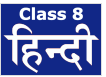Hindi for Class 8 (VIII) - CBSE and NCERT Curriculum

Includes 95 docs & 17 videos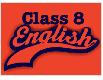English Honeydew for Class 8 - CBSE and NCERT Curriculum

Includes 51 docs96 videos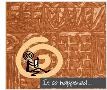English (It so Happened) for Class 8 - CBSE & NCERT

Includes 30 docs & 83 videosClass 8 Science by Full Circle

Includes 90 docs & 18 testsClass 8 Social Science by Full Circle

Includes 98 docs & 28 testsClass 8 Mathematics by Full Circle

90 docs & 16 tests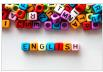Class 8 English by Full Circle

Includes 275 docs25 tests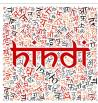कक्षा - 8 हिन्दी (Class 8 Hindi) by Full Circle

Includes 130 docs & 129 tests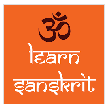कक्षा - 8 संस्कृत (Class 8 Sanskrit) by Full Circle

Includes 53 docs

The document How to prepare for Class 8 Maths: Tips & Tricks for Maths Class 8 Notes | EduRev is a part of Class 8 category.
All you need of Class 8 at this link: Class 8Use Code STAYHOME200 and get INR 200 additional OFF Use Coupon Code
All Tests, Videos & Notes of Class 8: Class 8

### Top Courses for Class 8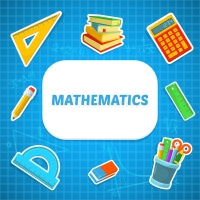### Top Courses for Class 8Track your progress, build streaks, highlight & save important lessons and more!

,

,

,

,

,

,

,

,

,

,

,

,

,

,

,

,

,

,

,

,

,

;